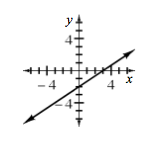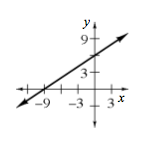### Home > A2C > Chapter 1 > Lesson 1.2.1 > Problem1-74

1-74.

Consider the equation $4x − 6y = 12$.

1. Predict what the graph of this equation looks like. Justify your answer.

Are there any exponents? What does this mean?

2. Solve the equation for $y$ and graph the equation.

Get y by itself.

−6y = −4x + 12

$y=\frac{2}{3}x-2$

$\frac{-6y}{-6}=\frac{-4x+12}{-6}$3. Explain clearly how to find the $x$- and $y$-intercepts.

The x-intercept is the point where $y$ = 0.
The y-intercept is the point where $x$ = 0.

4. Which form of the equation is best for finding intercepts quickly? Why?

It’s easier to use the given, standard form of the equation to find the intercepts quickly because there is minimal rewriting involved.

1. Find the $x$- and $y$-intercepts of $2x − 3y = −18$. Then use the intercepts to sketch a graph quickly.

Set $x = 0$ to find the $y$-intercept, and $y = 0$ to find the x-intercept.

$x$-intercept: $(−9, 0)$
$y$-intercept: $(0, 6)$